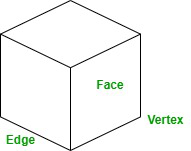GeeksforGeeks App
Open AppBrowser
Continue

# How many Vertices are there in a Cube?

The sub-discipline of mathematics that deals with the properties of two-dimensional or three-dimensional shapes are known as geometry. It is a form of visualized study of the shapes, structures, and their faces, points, line segments, etc.

Elucid is profoundly known as the father of geometry.

Geometry itself is divided into different types, that is

• Algebraic geometry
• Topology
• Non-Euclidean geometry
• Complex geometry
• Convex geometry
• Differential geometry and so on

Some Terminologies

• Line segment: Line segments are defined as the set of points in a line that lies between the two endpoints.
• Ray: Rays can be defined as a line with a single endpoint and the other point extended in opposite direction to infinity.
• Line: A line is a one-dimensional geometrical structure moving in opposite directions extending to infinity. A line only consists of length and does not have breadth or width.
• Point: A point is a zero-dimensional geometrical structure that is not composed of length, breadth, or height. Points are the base of any geometrical structure as all of them are formed by joining a set of points.

What are three-dimensional shapes?

The geometrical shapes that are composed of three dimensions length, breadth, and height or depth are known as three-dimensional shapes. These three-dimensional structures are observed in our daily life in the form of things present around us.

The five basic three-dimensional structures are cube, cuboid, cone, sphere, and cylinder.

What is a cube?

A cube is a three-dimensional shape symmetrical shape composed of 6 adjacent squares. A maybe be solid or hollow having six faces and 12 edges altogether.Cube

### How many vertices a cube does a cube have?

Vertices are the plural form of vertex which is the joining point of the edges of a shape. While considering a cube, is a symmetrical shape composed of six adjacent squares, having 12 edges and 8 vertices.

The number of vertices of a cube can simply be determined with a basic calculation.

Step 1: Add 2 to the number of edges present in a cube.

No. of edges = 12

12 + 2 = 14

Step 2: Subtract the number of faces present in a cube from the obtained answer.

No. of faces = 6

14 – 6 = 8

Hence, the number of vertices in a cube is 8.

### Sample Questions

Question 1. How many edges are there in the prism?

A triangular prism consists of 6 vertices. While a rectangular prism consists of 8 vertices.

Question 2. How many vertices are edges does a sphere has?

A sphere has no vertices or edges. While it only consists of one face.

Question 3. How many faces are there in a cylinder?

A cylinder is a three-dimensional shape with three faces.

Question 4. How many faces does a triangular prism have?